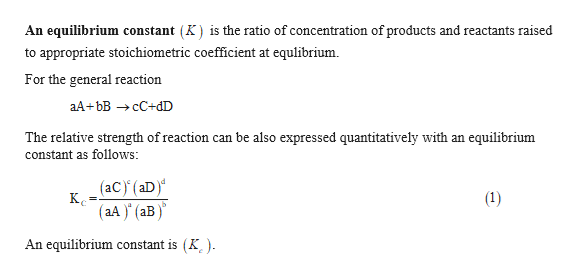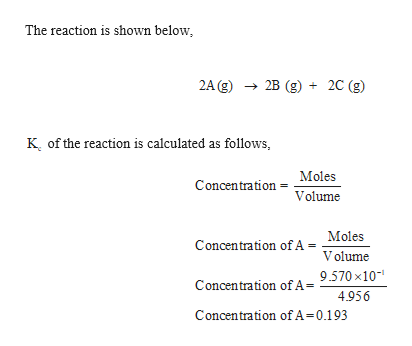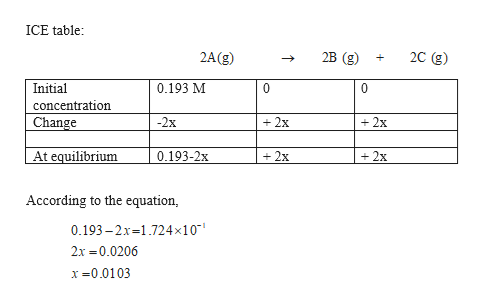A researcher is studying the decomposition of A as shown by the general reaction below:2 A(g) 2 B(g) + 2 C(g)Initially, the scientist fills an evacuated 4.956 L flask with 9.570 x 10-1 moles of species A. Upon equilibrium, it is determined that the concentration of A is 1.724 x 10-1 M. Calculate Kc.

Question

A researcher is studying the decomposition of A as shown by the general reaction below:

2 A(g) 2 B(g) + 2 C(g)

Initially, the scientist fills an evacuated 4.956 L flask with 9.570 x 10-1 moles of species A. Upon equilibrium, it is determined that the concentration of A is 1.724 x 10-1 M. Calculate Kc.

Step 1

Kc of the reaction is calculted using ICE table.help_outlineImage TranscriptioncloseAn equilibrium constant (K) is the ratio of concentration of products and reactants raised to appropriate stoichiometric coefficient at equlibrium For the general reaction aА + bв — сС+dD The relative strength of reaction can be also expressed quantitatively with an equilibrium constant as follows: (aC) (aD) К. (aA) (1) aB An equilibrium constant is (K ). fullscreen
Step 2

concentration of A is calculated.help_outlineImage TranscriptioncloseThe reaction is shown below 2A (g)2B (g) 2C (g) K of the reaction is calculated as follows, Moles Concentration Volume = Concentration ofA = Moles Volume Concentration of A = 9.570 x10 4.956 Concentration of A=0.193 fullscreen
Step 3

At equilibrium the concentration of...help_outlineImage TranscriptioncloseICE table 2C (g) 2B (g 2A(g) 0.193 M Initial 0 0 concentration +2x Change -2x +2x At equilibrium 0.193-2x 2x 2x According to the equation, 0.193-2x-1.724 x1 0 2x 0.0206 x0.0103 fullscreen

Want to see the full answer?

See Solution

Want to see this answer and more?

Our solutions are written by experts, many with advanced degrees, and available 24/7

See Solution
Tagged in

General Chemistry